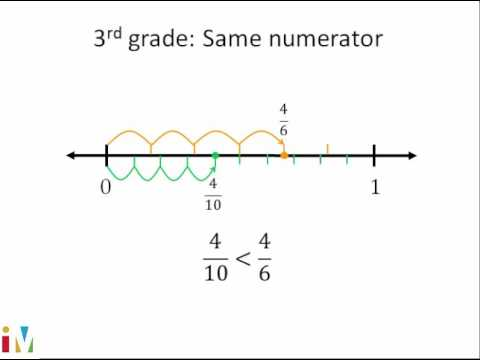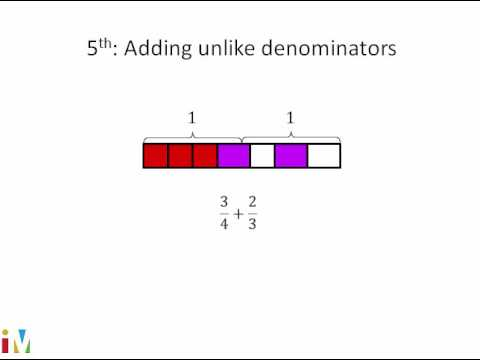# Intro to multiplying 2 fractions (Full video)

Description: This video will help you think about what it means to multiply two fractions. By drawing the problem and "seeing" the answer, you'll better understand how we arrived at it. Let's think about what it means to multiply 2 over 3, or 2/3, times 4/5. In the numerator, we just multiply the numerators. So it's going to be 3 times 5.

### Other videos you might be interested in### Comparing Fractions

#### Illustrative Mathematics